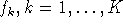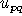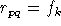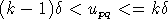Next: 9. Debugging Macros Up: A Tutorial on the Previous: 7. System Parameter Access with

# 8. Using Scalars and Floating Point Registers

Scalars have a wide-spread use throughout the entire EMME/2 system. They are accessible in the following ways:

Matrices:
Scalar matrices are fully integrated into all tools of the matrix editor.
Functions and expressions:
Scalars are accessible via the intrinsic function `ms()`, they can be used as ``programmable'' constants or to implement lookup tables.
Network:
Scalars can be accessed in network calculations by means of the `ms()` intrinsic. Summary results of network calculations can be saved into scalars, including sum, average, minimum/maximum value and element, number of elements processed and number of attribute values changed.
Macros:
Scalar substitution `%ms...%` can be used to insert scalar values, names and descriptions directly into dialog input and macro commands.

These possibilities make scalars the ideal vehicle to carry numeric values from one part of the system to another. Since, besides its numeric value, each scalar also has a name, description and a timestamp, they are also convenient places to store numeric results computed with a macro, along with their description.

By convention, the high-numbered macros `ms90`-`ms99` should be reserved for storing temporary values. This way, macros can freely use scalars in this range, without risking any conflict with application specific permanent scalars.

Before Release 8, the macro registers were limited to integer and text values, so that the explicit manipulation of scalars was the only means to perform floating computations within a macro. With Release 8, a set of 250 single precision floating point registers named `r1` to `r250` is introduced. These registers can be used in the same way as integer registers: they can be set to a value (`~r15=3.14159`), operated upon (`~r15+0.5`), tested (`~?rx<0`) and substituted (`%r15.5_10%`). With these floating point registers, many tasks which before had to be implemented using scalars just to do floating point operations can now be done more easily and efficiently.

In all commands that operate on floating point registers, the register number can either be specified directly (`r1` ...`r250`) or by using the current contents of one of the integer registers `x`, `y` or `z` (`rx`, `ry`, `rz`). With this indirection, a range of floating point registers can also be used as a vector which can easily be operated upon within index loops.

When working with floating point registers it is to be noted that, when switching from one module to the next or when invoking a lower level macro as a subroutine, their values are preserved by writing them (except those that are zero) to the external file `usemacro`. Even though this writing is done with a very high precision using the exponential format `e16.8`, the writing and reading may sometimes cause very tiny rounding errors, since there is no exact equivalence between the binary and decimal representation of fractional numbers. This does not apply to integer numbers up to 4'194'303 - these can be safely stored in floating point registers without any danger of rounding effects.

Another new enhancement that is introduced with Release 8 integrates the `get()/put()` stack of the EMME/2 expression evaluator into the macro language. The `get()/put()` stack are 250 words of auxiliary memory which are accessed from within expressions (e.g. functions, calculators) with the following intrinsic functions:

put(x):
The value of the the argument expression x is pushed on the stack and the stack pointer is incremented. The return value of the function is x.
get(i):
The value of the i-th element of the stack is returned. If i is not an integer, the truncated value is used as index.
puti(i):
The stack pointer is set the i, so that the next call to `put()` will write to the i-th element of the stack. If i is not an integer, the truncated value is used as index.

Note that the `get()/put()` stack is module specific, i.e. its values are set to zero when entering a new module and the final values are lost when the module is left.

Starting with Release 8 , the contents of the `get()/put()` stack can also be accessed --for both reading and writing-- directly from macros. This is done using the special floating point registers `g`N. These use exactly the same syntax as the standard floating point registers `r`N, but behave somewhate differently:

• Any change in the `g`N register will immadiately change the return value of the corresponding calls to `get()`.
• Any call to `put()` will immediately change the value of the corresponding register `g`N.
• At the start of a new module, the registers `g`N are initialized to zero.
• When a module is terminated the values of the `g`N registers are lost. (If needeed, bit 6 of the control register `o` can be used to ``collect'' stack values at the end of a module.)
• The `g`N registers are global registers, i.e. the same values are visible from all levels of macro invocation. Hence, it is possible to use these registers to pass return values from a lower level macro back to the calling macro (in this case, of course, it is your responsibility to make sure that there are no conflicts with any `get()/put()` calls used in the same module).

The `g`N registers allow an easy implementation of many tasks that, before, were not possible or very cumbersome to carry out, such as e.g. installing lookup tables for, or collecting side results of network and matrix calculations.

Exercise 8.1:
Write a macro `plotline` which takes a transit line name as parameter and determines the smallest network window covering the entire itinerary and then generates a plot with the transit volumes of this line.

Exercise 8.2:
Write a macro `speedist` which generates as output a table showing the distribution of the number of car kilometers traveled per speed intervals of 5 km/h.

Exercise 8.3:
Write a macro `mlookup` which contains the values of a lookup table () and performs a ``staircase lookup'' on a given matrixto obtain the result, where k is chosen such that, whereis an given interval size. Optional variant: Replace the staircase function by a continuous piecewise linear function.Next: 9. Debugging Macros Up: A Tutorial on the Previous: 7. System Parameter Access with

Heinz Spiess, EMME/2 Support Center
Fri Sep 27 13:04:28 MET DST 1996